# Parabolic coordinates

(diff) ← Older revision | Latest revision (diff) | Newer revision → (diff)

The numbersandrelated to rectangular Cartesian coordinatesandby the formulas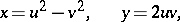whereand. The coordinate lines are two systems of mutually orthogonal parabolas with oppositely-directed axes.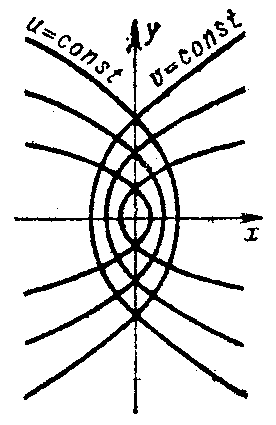Figure: p071170a

The Lamé coefficients (or scale factors) are given by: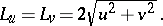The area element is given by:The fundamental operators of vector analysis are given by: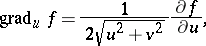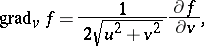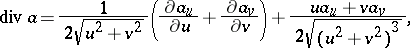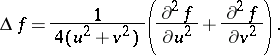In parabolic coordinates the Laplace equation allows separation of variables.

Using complex functions the coordinate transformation can be described by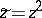, where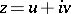and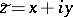.